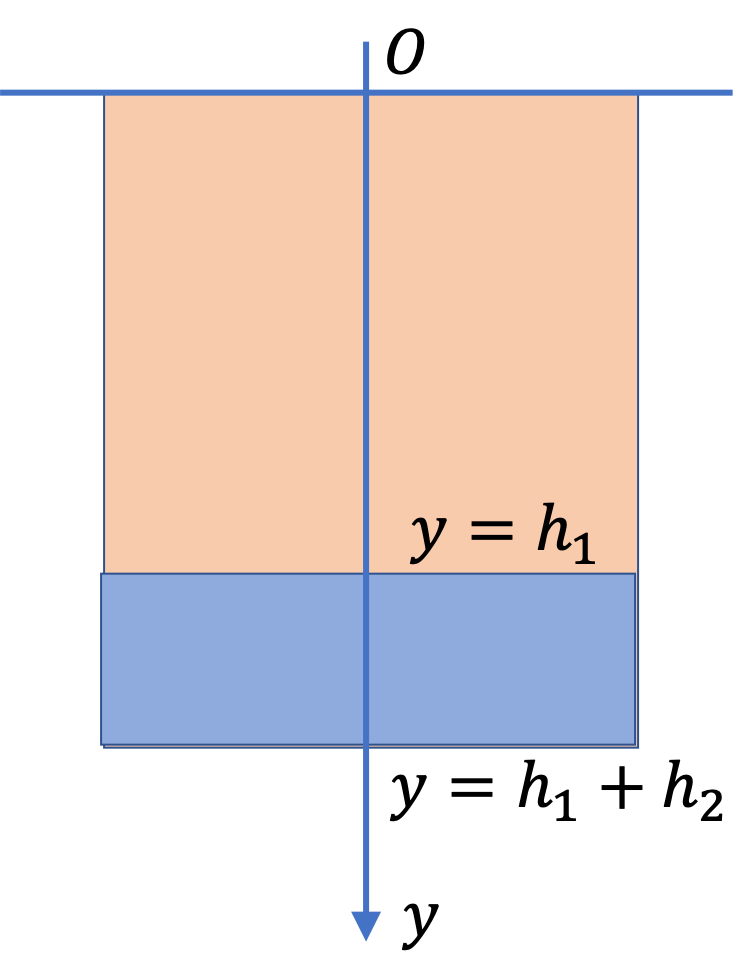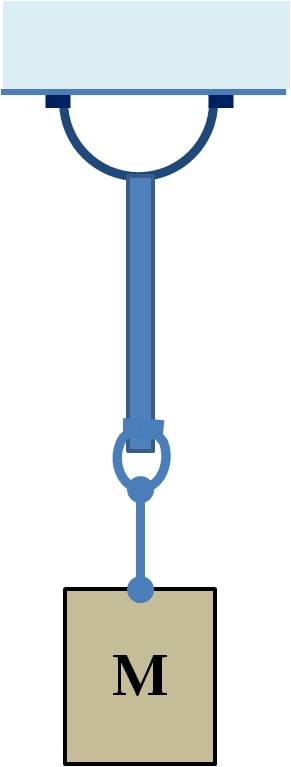## Section17.8Static Fluid Bootcamp

### Subsection17.8.7Miscellaneous

A tank is filled with water to $\frac{1}{4}$ of the height, and the rest is filled with oil of density $0.7\text{ g/cc}\text{.}$ How does the pressure in the tank vary with depth?

Hint

Use the $p(h) = p_0 + \rho g h$ formula. Your answer will be a stepwise function.

$p_0 + \rho_1 g y$ and $p_0 + \rho_1 g h_1 + \rho_2 g (y-h_1)$ depending on $y\text{.}$

Solution

We can use the $p(h) = p_0 + \rho g h$ formula to build the formula we need here. We use $p_0$ for the atmospheric pressure. Let $y$-axis point down with $y=0$ at the top of the oil layer. The formula for $p(y)$ here will be a step-wise function of $y\text{.}$Let $\rho_1$ and $\rho_2$ represent the densities of the oil and water respectively, and $h_1$ and $h_2$ are the heights of the liquids. Then we get the following for the pressure at various depths, as given by the $y$-coordinate, in the liquid.

\begin{align*} p \amp = p_0 + \rho_1 g y,\ \ 0 \le y \le h_1, \\ p \amp = p_0 + \rho_1 g h_1 + \rho_2 g (y-h_1),\ \ h_1 \le y \le h_1 + h_2. \end{align*}

How much weight can be supported by a $4\text{-in}$ diameter suction cup stuck to a flat glass ceiling in such a way that there is a perfect vacuum inside the suction cup.Hint

Balance weight of the block by the force due to pressure differece across the suction.

$335\text{ kg}.$

Solution

The weight of the block is balanced by the force due to pressure difference at the suction pump. Let $\Delta p$ be the pressure difference. This pressure acts on the perpendicular area equal to the area of the circle at the mouth of the suction pump. Therefore, the force by the pressure differece will be

\begin{equation*} F_\text{up} = \pi R^2 \Delta p, \end{equation*}

where $\Delta p$ is

\begin{equation*} \Delta p = p_{\text{out}} - p_{\text{in}}, \end{equation*}

where $p_{\text{out}}$ is the pressure outside the suction and $p_{\text{in}}$ inside. Balancing this force with the weight of the block we get

\begin{equation*} Mg - \pi R^2 \Delta p = 0, \end{equation*}

Solving for $M$ we get

\begin{equation*} M = \frac{\pi R^2 \Delta p}{g}. \end{equation*}

Putting in the numbers gives

\begin{align*} M \amp = \frac{\pi \left(4\times 2.54\times 10^{-2} \textrm{m} \right)^2 \times 1.013\times 10^{5}\ \textrm{Pa}}{9.81\ \textrm{m/s}^2}\\ \amp = 335\text{ kg}. \end{align*}

A test tube of radius $R$ is filled partially with a fluid and placed on a rotor so that the axis of rotation passes through the center of the tube as shown in Figure 17.8.32. When the rotor spins the tube ata constant angular speed of $\omega\text{,}$ the surface of fluid in the tube is no longer horizontal. Find the shape of the surface expressed as $z$ as a function of $r\text{,}$ where $r=\sqrt{x^2 + y^2}\text{.}$

Hint

Examine forces on a mass element at the edge of the surface. For radial direction, use uniform circular motion. For other directions, balance forces.

$z = \frac{\omega^2}{2g}\; r^2\text{.}$

Solution

To find the shape of the surface, we will examine the dynamics of a small element of mass $dm=\rho dx dy dz$ at coordinate $(x,y,z)$ located at the surface of the spinning fluid as shown in Figure 17.8.33.

Let $P$ denote the pressure immediately behind the fluid element. Two forces act on this element, viz., weight and the force by pressure, which will be directed prependicular to the surface. As a result of these forces, the element has a uniform circular motion of radius $r=\sqrt{x^2+y^2}\text{.}$

From symmetry of the situation shown in Figure 17.8.33, it is sufficient to work with point $(x,y,z)=(0,y,z)\text{,}$ and in the end replace $|y|$ by $r\text{.}$

To find the components of the force by pressure of the fluid, we will need to find direction of this force. This force will be pointed perpendicular to the surface at that point, similar to what we expect from a normal force. To find this direction, we need to first find the tangential direction of the curve in the $yz$-plane.

Let $z(y)$ be the curve in the $yz$-plane. The direction of the tangent, as given by the unit vector $\hat t\text{,}$ will be

\begin{equation*} \hat t = b \left( \hat j + \left( \frac{dz}{dy} \right)\hat k \right), \end{equation*}

where

\begin{equation*} b = 1/\sqrt{1 + (dz/dy)^2}. \end{equation*}

From this we get the direction of the force by pressure, to be denited by $\hat u\text{,}$ will be

\begin{equation*} \hat u = b\left( - \left( \frac{dz}{dy}\right)\hat j + \hat k \right). \end{equation*}

Therefore, force by pressure behind the element is

\begin{equation*} \vec F = PdA\;\hat u = b PdA \left[ - \left( \frac{dz}{dy}\right)\hat j + \hat k\right]. \end{equation*}

The other force is the weight

\begin{equation*} \vec W = -dm\; g \;\hat k. \end{equation*}

Net force, $\vec F_\text{net} = \vec F + \vec W = m\vec a\text{,}$ where $\vec a$ is the centripetal acceleration, which for radius $y$ and angular speed $\omega$ will be

\begin{equation*} \vec a = - \omega^2 y \hat j. \end{equation*}

Therefore, we will get equations along $y$ and $z$ axis will be

\begin{align*} - b\amp P dA \left( \frac{dz}{dy}\right) = -dm\; \omega^2 y.\\ b\amp PdA - dm\; g = 0. \end{align*}

Eliminating $PdA$ from these two gives

\begin{equation*} \frac{dz}{dy} = \frac{\omega^2}{g} y. \end{equation*}

This differential equation has a simple solution.

\begin{equation*} z = \frac{\omega^2}{2g}\; y^2 + c, \end{equation*}

where $c$ is constant of integration. Since, $y=0,z=0$ is on the curve, we have $c=0\text{.}$ Thus,

\begin{equation*} z = \frac{\omega^2}{2g}\; y^2. \end{equation*}

Replacing $|y|$ by $r$ we get

\begin{equation*} z = \frac{\omega^2}{2g}\; r^2. \end{equation*}

A piece of plastic floats in water of density $1.0\text{ g/cc}$ with $90\%$ of its volume submerged. An oil of density $0.7\text{ g/cc}$ is poured over the water. It is found that less volume of plastic piece than earlier is now submerged in water. Find the fraction of volume of the plastic piece under water when it is completely submerged in oil.

Hint

Buoyancy now will be sum of buoyancies from oil and water layers.

$\frac{1}{3}\text{.}$

Solution

The $90\%$ submersion in water gives the density of plastic to be

\begin{equation*} \rho_\text{pl} = 0.90\text{ g/cc}. \end{equation*}

Now, we look at the situation when oil is poured over water till the top of the oil layer is level with the top of the block. Now, the buoyancy will be from pressure at the bottom of the box. Balancin force of buouancy with the wight of the block we get

\begin{equation*} \left( A(h-d)\rho_\text{ol} + Ad\rho_\text{wt} \right)\; g = A h \rho_\text{pl}\;g. \end{equation*}

Let $x=d/h$ to simplify expression. Also, let us write $a=\rho_\text{wt}/\rho_\text{pl}\text{,}$ and $a=\rho_\text{wt}/\rho_\text{pl}\text{.}$ Now, this equation simplifies to

\begin{equation*} (1-x) b + a x = 1. \end{equation*}

Therefore,

\begin{equation*} x = \frac{1-b}{a-b} = \frac{\rho_\text{pl} - \rho_\text{ol} }{ \rho_\text{wt} - \rho_\text{ol} }. \end{equation*}

With given numerical values we get

\begin{equation*} x = \frac{0.8 - 0.7}{1.0 - 0.7} = \frac{1}{3}. \end{equation*}

A copper ball of mass $2\text{ kg}$ is hung from a support, and dipped in water as shown in Figure 17.8.37. The arrangement is put in an elevator. (a) What will be the tension in the string when the elevator has an upward accelerating of magnitude $2 \text{ m/s}^2$ ? (b) What will be the tension in the string when the elevator has a downward acceleration of magnitude $2 \text{ m/s}^2\text{?}$

Density of copper = $8,960\text{ kg/m}^3$ and density of water = $1,000\text{ kg/m}^3\text{.}$

Hint

Set up $\vec F=m\vec a$ for the ball.

(a) $25.81\text{ N}\text{,}$ (b) $17.81\text{ N}\text{.}$

Solution 1 (a)

The free-body diagram of the ball is shown in Figure 17.8.38. Let $V$ denote the volume of the ball, $\rho$ denote density of copper, and $\rho_0$ denote density of water. Equation of motion for upwardly directed acceleration $a$ is

\begin{equation*} T + F_B - M g = M a, \end{equation*}

with $M=V\rho$ and $F_B=V\rho_0\;g\text{.}$

From $M=V\rho\text{,}$ we get $V\text{,}$ which we use in $F_B$ to get

\begin{equation} T = M (a+g) - (M/\rho)\rho_0\;g.\label{eq-pr-ball-in-fluid-in-elevator-a}\tag{17.8.1} \end{equation}

Now, using numerical values we get

\begin{align*} T \amp = 2(2 + 9.81) - (2/8,960)\times 1000\times 9.81\\ \amp = 23.62+2.19 = 25.81\text{ N}. \end{align*}
Solution 2 (b)

In Eq. (17.8.1), now, we have $a$ negative. This will give

\begin{align*} T \amp = 2(-2 + 9.81) - (2/8,960)\times 1000\times 9.81\\ \amp = 15.62+2.19 = 17.81\text{ N}. \end{align*}

A piece of wood of uniform cross-sectional area floats over water with a height $h_0$ submerged below water level. It is pushed sligltly into the water, and let go from rest. Show that the subsequent motion of the block is a simple harmonic oscillation, and find the frequency of oscillations.

Hint

Follow the motion of the center of mass of the wooden piece.

$\frac{1}{2\pi}\;\sqrt{ \frac{g }{h_0} }\text{.}$

Solution

For simplicity consider a rectangular shaped box as shown in Figure 17.8.40.

We need to analyze two situations, one at the equilibrum Fig. (a) and another at an arbitrary instant during the motion of the block, Fig (b). As shown in the figure, with positive $y$ axis pointed down we get the following equaiton from the quilibrium situation.

\begin{equation} mg - A h_0 \rho_w g = 0,\label{eq-pr-smh-wood-bouncing-in-water-equilib}\tag{17.8.2} \end{equation}

which gives $m=A h_0 \rho_w\text{.}$ From the situation at arbitrary instant, we get

\begin{equation} mg - A (h_0+y) \rho_w g = m a_y = m \frac{d^2y}{dt^2}.\label{eq-pr-smh-wood-bouncing-in-water-non-equilib}\tag{17.8.3} \end{equation}

From these equations, we can get

\begin{equation} \frac{d^2y}{dt^2} = -\frac{A y\rho_w g }{m}y.\tag{17.8.4} \end{equation}

Using expresson of $m$ here gives

\begin{equation} \frac{d^2y}{dt^2} = -\frac{g }{h_0}y.\tag{17.8.5} \end{equation}

This is an equation of a simple harmonic motion with angular frequency, $\omega$ given by

\begin{equation*} \omega = \sqrt{ \frac{g }{h_0} }. \end{equation*}

Frequency will be this divided by $2\pi\text{.}$

\begin{equation*} f = \frac{1}{2\pi}\;\sqrt{ \frac{g }{h_0} }. \end{equation*}